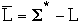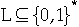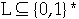##CS 660: Combinatorial Algorithms More NP Completness

[To Lecture Notes Index]
San Diego State University -- This page last updated November 29, 1995## Reference

Introduction to Algorithms, by Cormen, Leiserson, Rivest, Chapter 36

## NP Completeness

Abstract problem Q
Q is a binary relation on set of problem instances I and a set of problem solutions S

Example - Shortest Path
Find the shortest path in a graph between two vertices.
I = { (G, s, e) | G = (V, E) is unweighted undirected graph, s propersubset ofV is the start vertex, e propersubset ofV is the end vertex }
S = { (G, p) | G = (V, E), p is a path in G }
Let a =< G, s, e > propersubset ofI then
Q(a) = { (G, p) |p is the shortest path between s and e in G}
Usually use Shortest Path instead of Q

Decision Problems - Problems with yes/no answers

Given a graph G = (V, E) and two vertices s, e propersubset ofV does there exist a path in G between s and e whose length is at most k?
I = { (G, s, e, k) | G = (V, E) is unweighted undirected graph, s propersubset ofV is the start vertex, e propersubset ofV is the end vertex, k upper bound on shortest path }
S = { 0, 1 }

Let a =< G, s, e, k > propersubset of I then
Path(a) = 1 if shortest path from s to e has length <= k
Path(a) = 0 if shortest path from s to e has length > k
Decision problems simplify theory

Most problems can be restated as a decision problem

Concrete Problem is a problem whose instance is the set of binary strings

Example:
Let x be an integer, is x even?
Let I = { binary strings}, S = { 0, 1 }
Let x propersubset of I then we have
Even(x) = 1
if x1 = 1
Even(x) = 0
if x0 = 1

An algorithm solves a concrete problem in time O(T(n)) if
given a propersubset of I with n = |a| the algorithm finds the solution in at most O(T(n)) time

Polynomial-time solvable
A concrete problem is polynomial-time solvable if there exits an algorithm to solve it in time O(nk) for some constant k

Complexity class P = { concrete problem that are polynomial-time solvable }
Encodings
An encoding "e" is a mapping from a set S to binary strings
Use encodings to map abstract problems to concrete problemsExample - Shortest Path```              s             a             b             c             d             e
s             0             1             0             1             0             0
a             1             0             1             0             1             0
b             0             1             0             1             0             1
c             1             0             1             1             0             0
d             0             1             0             1             0             1
e             0             0             1             0             1             0

```

010100101010010101101100010101001010
Encodings change the time complexity

Example Is K a prime number?
Algorithm
divide K by all integers 2, 3, 4, ..., K -1
If any integer divides K it is not prime
Worst case requires K -2 divisions (Use K for simplicity)
Embedding 1 K -> binary representation of K
Input length is n = lg(K)
Time Complexity T(n) = K = 2n
Embedding 2 Place the J'th bit of K in location 2J, fill rest of locations with 0.
Input length is n = K
Time Complexity T(n) = n
f : {0, 1}* -> {0, 1}* is polynomial-time computable if there exists a polynomial-time algorithm A that, given any input x propersubset of {0, 1}*, produces as output f(x)

Given set of problem instances I, encodings e1 and e2 are polynomially related if there exists polynomial-time computable functions f1 and f2 such that

a propersubset of I -> f1(e1(a)) = e2(a) and f2(e2(a)) = e1(a)

Lemma
Let Q be an abstract decision problem on an instance set I, and let e1 and e2 be encodings polynomially related encodings on I. Then
e1(Q) propersubset of P if and only if e2(Q) propersubset of P

The Final Step - Formal-language

Alphabet Sigma a finite set of symbols

Language L over Sigma
any set of strings made up of symbols from Sigma

Empty string denoted by [[epsilon]]

Empty language by ø

Sigma* language of all strings over Sigma

Complement of LConcatenation of two languages L1 and L2 is the language:
L = { xy : x propersubset ofL1 and y propersubset of L2}
Closure or Kleene Star of L is
L*={[[epsilon]]} [[union]] L [[union]] L1 [[union]] L2[[union]] L3 [[union]] L4[[union]] ...
Decision Problems as a language
Let Sigma = {0, 1} then set of binary strings = Sigma*
Let Q be a decision problem
L = {x propersubset ofSigma* : Q(x) = 1 }

Algorithm A accepts a string x propersubset of{ 0, 1 }* if A(x) = 1

Algorithm A rejects a string x propersubset of{ 0, 1 }* if A(x) = 0

The language accepted by algorithm A is
L = { x propersubset of{ 0, 1 }* : A(x) = 1}

A language L is decided by algorithm A if every binary string is either accepted or rejected by algorithm A

A language L is accepted in polynomial time by algorithm A if for any string of length n in L is accepted by A in time O(nk)

A language L is decided in polynomial time by algorithm A if for any string of length n in { 0, 1 }* is decided by A in time O(nk)

We can redefine P as:
P = {: there exists an algorithm A that decides L in polynomial time }

Theorem
P = { L : L is accepted by a polynomial-time algorithm }
Polynomial-time Verification

Hamiltonian cycle of an undirected graph G= (V, E) is a simple cycle that contains each vertex in V

A hamiltonian graph is a graph that has a hamiltonian cycle.

Let m = |V|, it takes m! operations to determine if G= (V, E) is a hamiltonian graph

If Xiaojun claims a graph is hamiltonian graph and provides a the vertices in order on the hamiltonian cycle then we can verify his claim in polynomial time

The potential cycle is called the certificate.

A verification algorithm is a two-argument algorithm A

One argument is an ordinary input string x, the other is the certificate a binary string y.

"A" verifies input x if there exists a certificate such that
A(x, y) = 1

The language verified by A is:

L = { x propersubset of{ 0, 1 }* : there exists y propersubset of{ 0, 1 }* such that A(x, y) = 1}
Complexity Class NP

Complexity class NP is the class of languages that can be verified by a polynomial-time algorithm

Theoremproof:
If L propersubset ofP then L is accepted in polynomial time by algorithm B
That is for any x propersubset ofL we can compute B(x) in polynomial time and B(x) = 1
Now let A(x,y) = B(x)
Then A(x, y) verifies x in polynomial time
Language L1 is polynomial-time reducible to language L2 if there exists a polynomial-time computable function
f : { 0, 1 }* -> { 0, 1 }*
such that for all x propersubset of{ 0, 1 }* we have:
x propersubset ofL1 if and only if f(x) propersubset ofL2

We write L1 <=p L2

Lemma 36.3 Assume that L1 <=p L2, then L2 propersubset ofP implies L1 propersubset ofP

A languageis NP-complete if

1. L propersubset of NP

2. L1 <=p L for all L1 propersubset of NP

Theorem 36.4
a. If any NP-complete problem is polynomial solvable then
P = NP.
b. If any NP-complete problem is not polynomial solvable then all NP-complete problems are not polynomial solvable